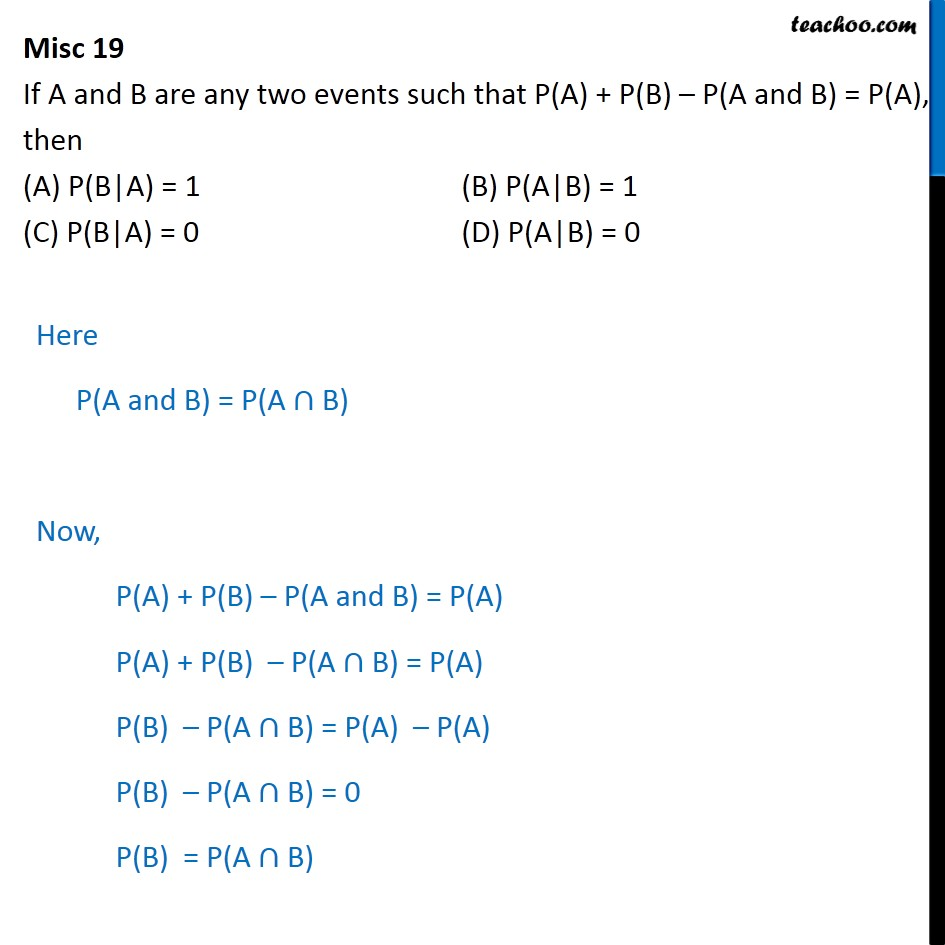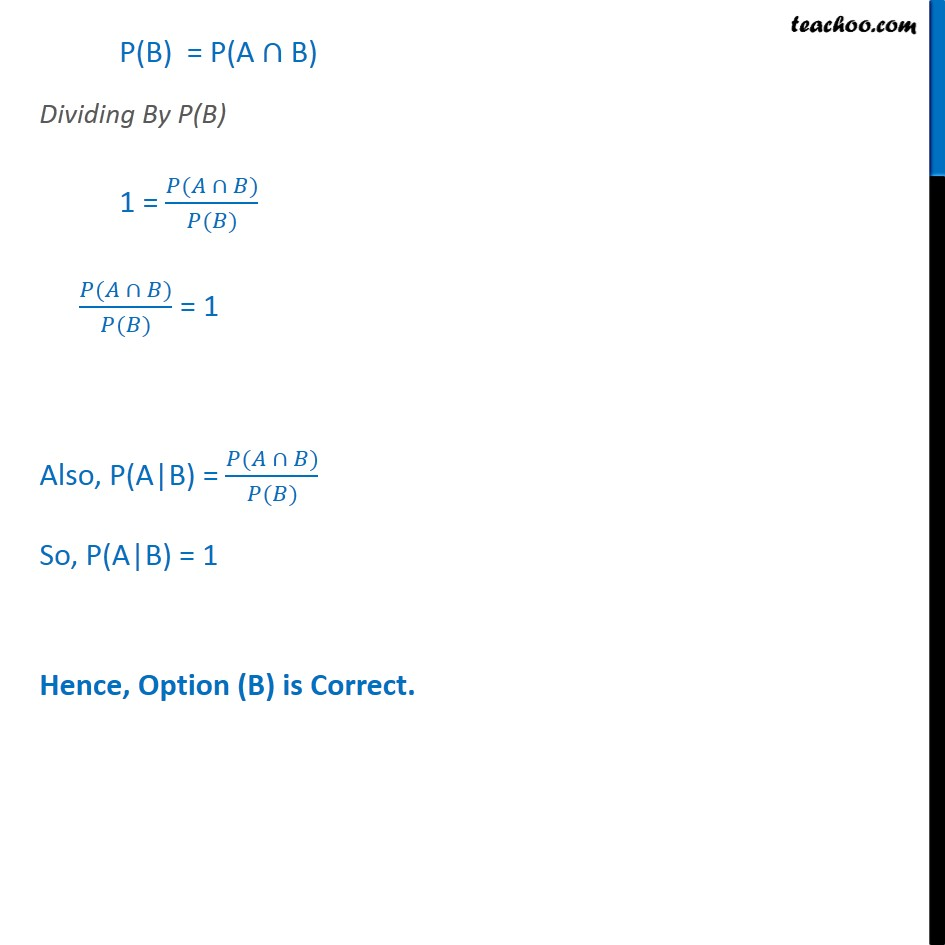Conditional Probability - Values given

Chapter 13 Class 12 Probability
Concept wiseIntroducing your new favourite teacher - Teachoo Black, at only ₹83 per month

### Transcript

Misc 19 If A and B are any two events such that P(A) + P(B) P(A and B) = P(A), then (A) P(B|A) = 1 (B) P(A|B) = 1 (C) P(B|A) = 0 (D) P(A|B) = 0 Here P(A and B) = P(A B) Now, P(A) + P(B) P(A and B) = P(A) P(A) + P(B) P(A B) = P(A) P(B) P(A B) = P(A) P(A) P(B) P(A B) = 0 P(B) = P(A B) P(B) = P(A B) Dividing By P(B) 1 = ( ) ( ) ( ) ( ) = 1 Also, P(A|B) = ( ) ( ) So, P(A|B) = 1 Hence, Option (B) is Correct.